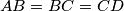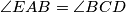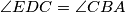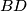### IMO Shortlist 2017 problem G1

Kvaliteta:
Avg: 0,0
Težina:
Avg: 6,0

Let$ABCDE$ be a convex pentagon such that$AB=BC=CD$,$\angle{EAB}=\angle{BCD}$, and$\angle{EDC}=\angle{CBA}$. Prove that the perpendicular line from$E$ to$BC$ and the line segments$AC$ and$BD$ are concurrent.

Izvor: https://www.imo-official.org/problems/IMO2017SL.pdf